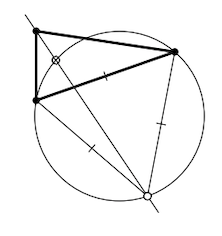Skip to main content
$$\newcommand{\id}{\mathrm{id}}$$ $$\newcommand{\Span}{\mathrm{span}}$$ $$\newcommand{\kernel}{\mathrm{null}\,}$$ $$\newcommand{\range}{\mathrm{range}\,}$$ $$\newcommand{\RealPart}{\mathrm{Re}}$$ $$\newcommand{\ImaginaryPart}{\mathrm{Im}}$$ $$\newcommand{\Argument}{\mathrm{Arg}}$$ $$\newcommand{\norm}{\| #1 \|}$$ $$\newcommand{\inner}{\langle #1, #2 \rangle}$$ $$\newcommand{\Span}{\mathrm{span}}$$

# 9.5: Arcs of circlines

$$\newcommand{\vecs}{\overset { \rightharpoonup} {\mathbf{#1}} }$$ $$\newcommand{\vecd}{\overset{-\!-\!\rightharpoonup}{\vphantom{a}\smash {#1}}}$$$$\newcommand{\id}{\mathrm{id}}$$ $$\newcommand{\Span}{\mathrm{span}}$$ $$\newcommand{\kernel}{\mathrm{null}\,}$$ $$\newcommand{\range}{\mathrm{range}\,}$$ $$\newcommand{\RealPart}{\mathrm{Re}}$$ $$\newcommand{\ImaginaryPart}{\mathrm{Im}}$$ $$\newcommand{\Argument}{\mathrm{Arg}}$$ $$\newcommand{\norm}{\| #1 \|}$$ $$\newcommand{\inner}{\langle #1, #2 \rangle}$$ $$\newcommand{\Span}{\mathrm{span}}$$ $$\newcommand{\id}{\mathrm{id}}$$ $$\newcommand{\Span}{\mathrm{span}}$$ $$\newcommand{\kernel}{\mathrm{null}\,}$$ $$\newcommand{\range}{\mathrm{range}\,}$$ $$\newcommand{\RealPart}{\mathrm{Re}}$$ $$\newcommand{\ImaginaryPart}{\mathrm{Im}}$$ $$\newcommand{\Argument}{\mathrm{Arg}}$$ $$\newcommand{\norm}{\| #1 \|}$$ $$\newcommand{\inner}{\langle #1, #2 \rangle}$$ $$\newcommand{\Span}{\mathrm{span}}$$

A subset of a circle bounded by two points is called a circle arc.

More precisely, suppose $$A, B, C$$ are distinct points on a circle $$\Gamma$$. The circle arc $$ABC$$ is the subset that includes the points $$A, C$$ as well as all the points on $$\Gamma$$ that lie with $$B$$ on the same side of $$(AC)$$.

For the circle arc $$ABC$$, the points $$A$$ and $$C$$ are called endpoints. There are precisely two circle arcs of $$\Gamma$$ with the given endpoints; they are opposite to each other.

Suppose $$X$$ be another point on $$\Gamma$$. By Corollary 9.3.2 we have that $$2 \cdot \measuredangle AXC \equiv 2 \cdot \measuredangle ABC$$; that is,

$$\measuredangle AXC \equiv \measuredangle ABC$$     or     $$\measuredangle AXC \equiv \measuredangle ABC + \pi.$$Recall that $$X$$ and $$B$$ lie on the same side from $$(AC)$$ if and only if $$\angle AXC$$ and $$\angle ABC$$ have the same sign (see Exercise 3.4.2). It follows that

• $$X$$ lies on the arc $$ABC$$ if and only if

$$\measuredangle AXC \equiv \measuredangle ABC$$;

• $$X$$ lies on the arc opposite to $$ABC$$ if

$$\measuredangle AXC \equiv \measuredangle ABC + \pi$$.

Note that a circle arc $$ABC$$ is defined if $$\triangle ABC$$ is not degenerate. If $$\triangle ABC$$ is degenerate, then arc $$ABC$$ is defined as a subset of line bounded by $$A$$ and $$C$$ that contain $$B$$.More precisely, if $$B$$ lies between $$A$$ and $$C$$, then the arc $$ABC$$ is defined as the line segment $$[AC]$$. If $$B'$$ lies on the extension of $$[AC]$$, then the arc $$AB'C$$ is defined as a union of disjoint half-lines $$[AX)$$ and $$[CY)$$ in $$(AC)$$. In this case the arcs $$ABC$$ and $$AB'C$$ are called opposite to each other.In addition, any half-line $$[AB)$$ will be regarded as an arc. If $$A$$ lies between $$B$$ and $$X$$, then $$[AX)$$ will be called oppostie to $$[AB)$$. This degenerate arc has only one endpoint $$A$$.

It will be convenient to use the notion of circline, that means circle or line. For example any arc is a subset of a circline; we also may use the term circline arc if we want to emphasise that the arc might be degenerate. Note that for any three distinct points $$A, B$$, and $$C$$ there is a unique circline arc $$ABC$$.

The following statement summarizes the discussion above.

Proposition $$\PageIndex{1}$$

Let $$ABC$$ be a circline arc and $$X$$ be a point distinct from $$A$$ and $$C$$. Then

(a) $$X$$ lies on the arc $$ABC$$ if and only if

$$\measuredangle AXC = \measuredangle ABC;$$

(b) $$X$$ lies on the arc opposite to $$ABC$$ if and only if

$$\measuredangle AXC \equiv \measuredangle ABC + \pi$$;

Exercise $$\PageIndex{1}$$

Given an acute triangle $$ABC$$ make a compass-and-ruler construction of the point $$Z$$ such that

$$\measuredangle AZB = \measuredangle BZC = \measuredangle CZA = \pm \dfrac{2}{3} \cdot \pi$$

HintGuess a construction from the diagram. To show that it produces the needed point, apply Theorem 9.2.1.

Exercise $$\PageIndex{2}$$

Suppose that point $$P$$ lies on the circumcircle of an equilateral triangle $$ABC$$ and $$PA \le PB \le PC$$. Show that $$PA + PB = PC$$.

HintShow that $$P$$ lies on the arc opposite from $$ACB$$; conclude that $$\measuredangle APC = \measuredangle CPB = \pm \dfrac{\pi}{3}$$.

Choose a point $$A' \in [PC]$$ such that $$PA' = PA$$. Note that $$\triangle APA'$$ is equilateral. Prove and use that $$\triangle AA'C \cong \triangle APB.$$

A quadrangle $$ABCD$$ is inscribed if all the points $$A, B, C$$, and $$D$$ lie on a circline $$\Gamma$$. If the arcs $$ABC$$ and $$ADC$$ are opposite, then we say that the points $$A, B, C$$, and $$D$$ appear on $$\Gamma$$ in the same cyclic order.

This definition makes it possible to formulate the following refinement of Corollary 9.3.2 which includes the degenerate quadrangles. It follows directly from Proposition $$\PageIndex{1}$$.

Proposition $$\PageIndex{2}$$

A quadrangle $$ABCD$$ is inscribed in a circline if and only if

$$\measuredangle ABC = \measuredangle ADC$$     or     $$\measuredangle ABC \equiv \measuredangle ADC + \pi.$$

Moreover, the second identity holds if and only if the points $$A,B,C,D$$ appear on the circline in the same cyclic order.